# Lens

Lens :- A lens is a piece of transparent material with two refracting surfaces such that at least one is curved and refractive index of its material is different from that of the surroundings.

Types of lens :- The two main types of lenses are

• Convex Lens (Converging)
• Concave Lens (Diverging)

Convex Lens (Converging Lens)

A thin spherical lens with refractive index greater than that of surroundings behaves as a convergent or convex lens, i.e., converges parallel rays(i.e. paraxial) if its central portion is thicker than marginal one.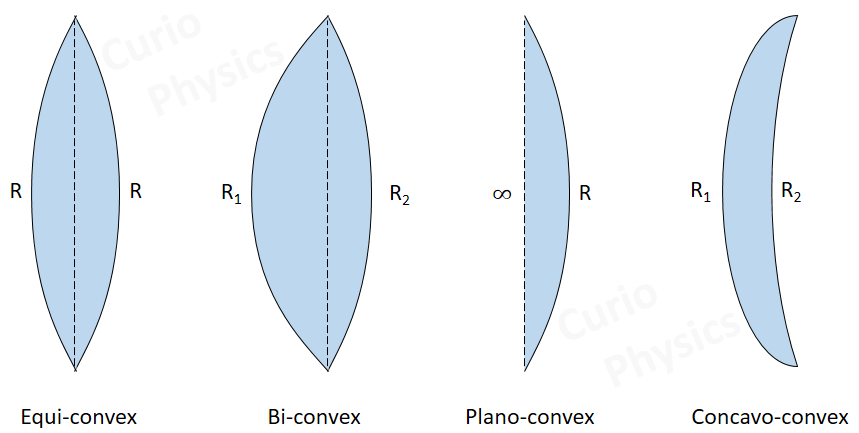Concave Lens (Diverging)

If the central portion of a lens is thinner than marginal, it diverges parallel rays and behaves as divergent or concave lens.Technical Terms Related to lens :-

(a) Centers of curvature (C) :-

• A lens has two spherical surfaces. Each of these surfaces forms a part of a sphere. The centers of these spheres are called centers of curvature of the lens.
• The center of curvature of a lens is usually represented by the letter C. Since there are two centers of curvature, we may represent them as C1 and C2.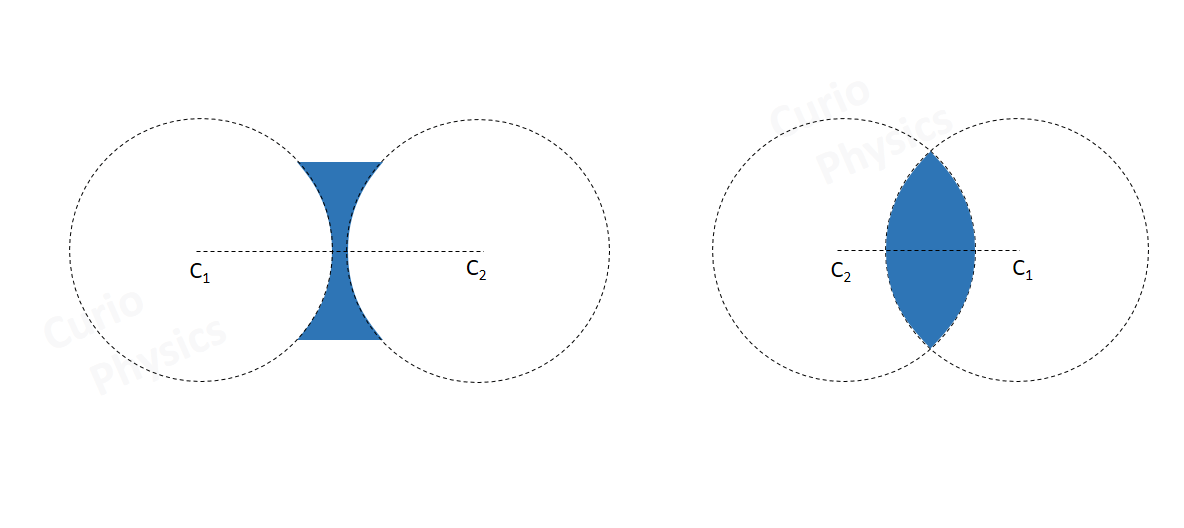(b) Optical Centre (O) :-  The central point of a lens is its optical center. For a given lens light through optical center passes undeviated. It is usually represented by the letter O.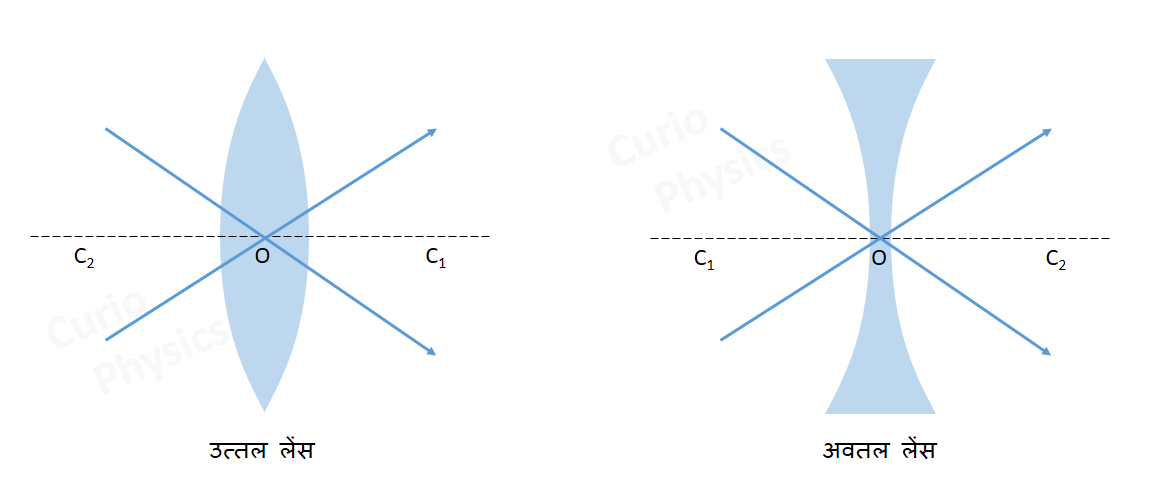(c) Principal Axis :- Principal axis is the line (C1 C2) joining the centers of curvature of the two surfaces of the lens.

(d) Principal Focus :- A lens has two surfaces and hence two focal points.

First principal focus – The point on which the light rays coming parallel to the principal axis after refraction from the lens meet(convex lens) or appear to diverge (concave lens) on the principal axis is called the first principal focus of the lens. It is denoted by F1.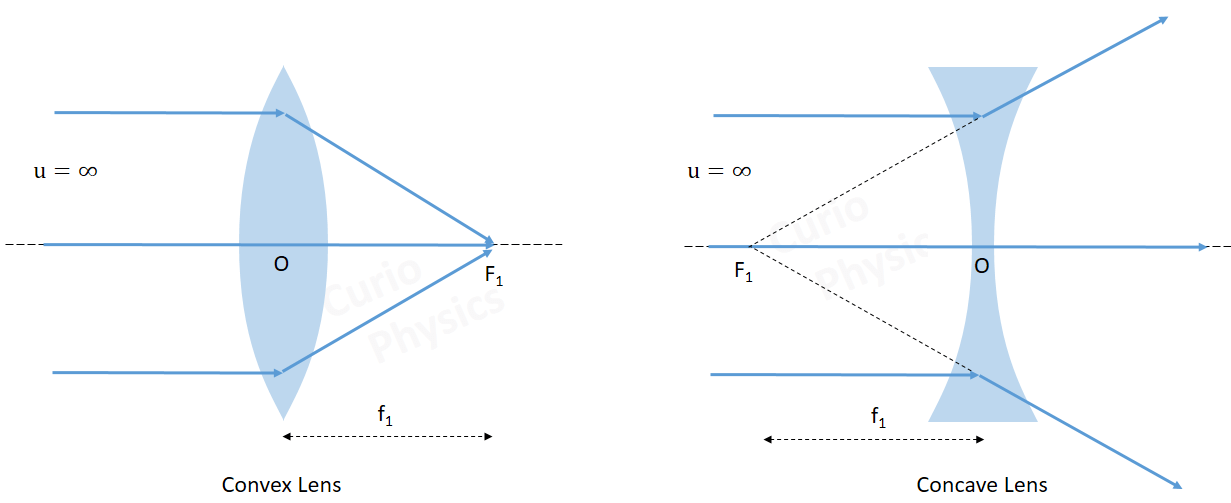Second principal focus of a lens is that point on the principal axis of the lens at which if an object is placed, the image would be formed at infinity(convex lens) or the light rays directed towards the point will meet at infinity(concave lens).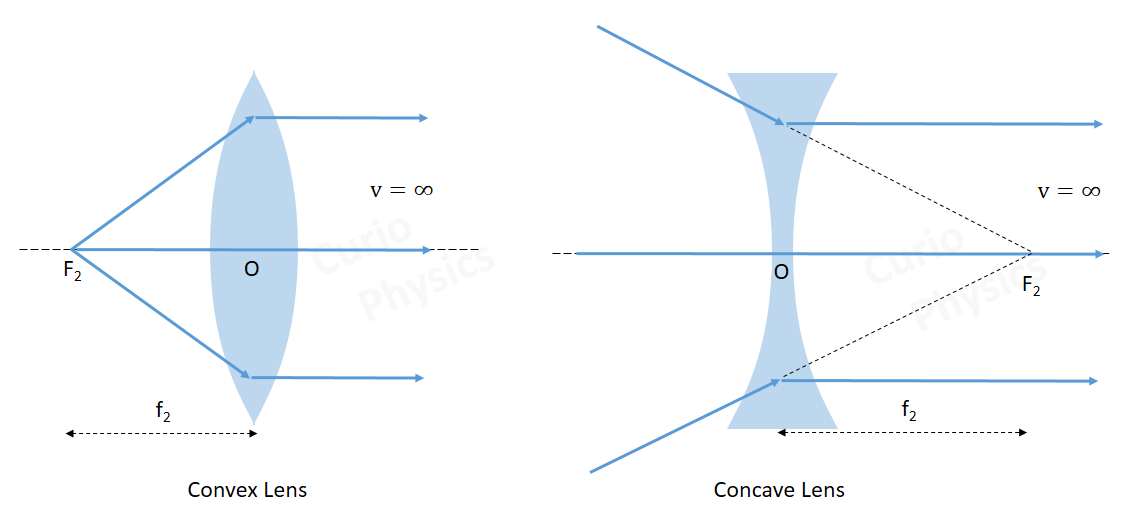(e) Focal Length (f) :- Focal Length (f) is defined as the distance between optical center of a lens and the point where the parallel beam of light converges (convex lens) or appears to diverge (concave lens).

So for convex lens focal length f = positive and for concave lens focal length f = negative.

(f) Aperture :- In reference to a lens, aperture means the effective diameter of the lens.

Intensity of image formed by a lens which depends on the light passing through the lens will depend on the square of aperture, i.e., Intensity ∝ (Aperture)2

Next Topic :- Lens maker’s formula